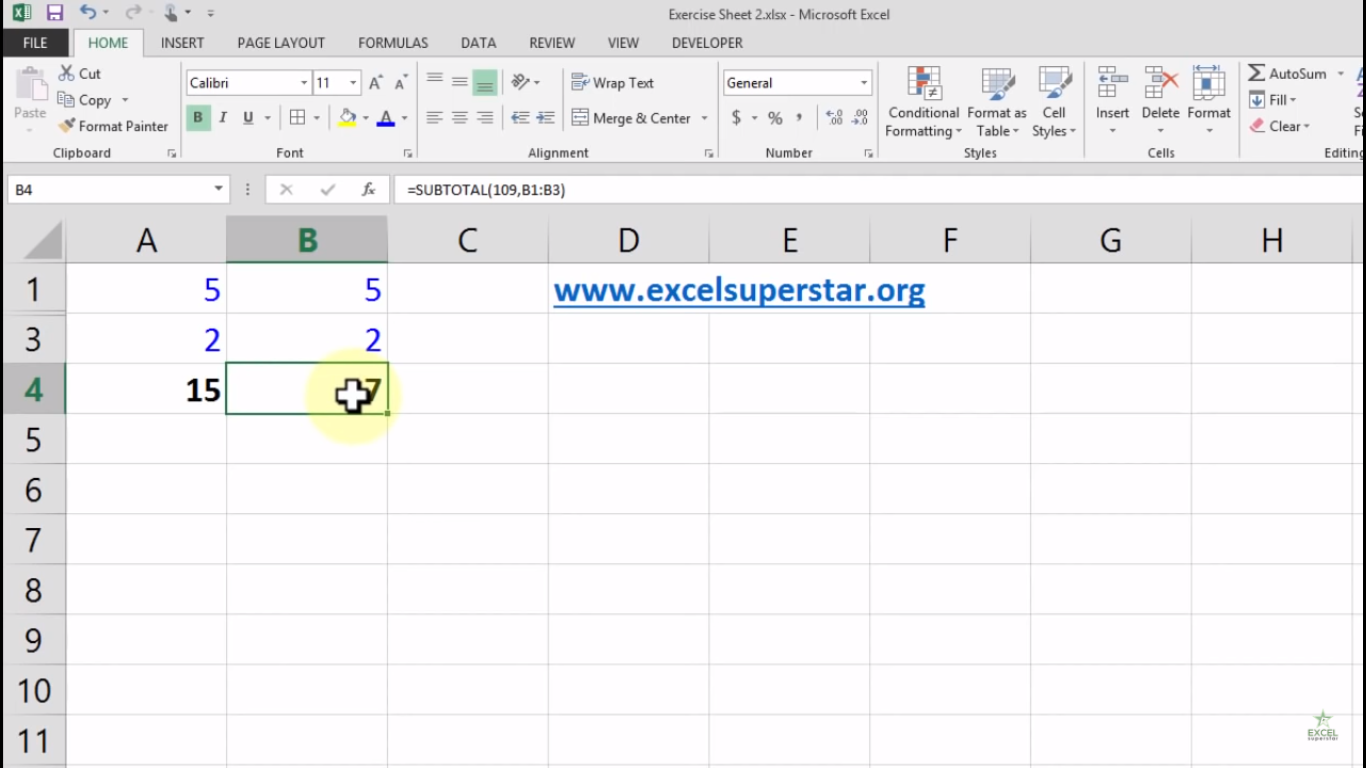# Excel SUBTOTAL FunctionIn this blog, we will learn about How to use SUBTOTAL Function in Excel

Use the Subtotal Function in Excel instead of using SUM, COUNT, MAX, etc to ignore rows are hidden by a filter or to ignore manually hidden rows

1. Rows Hidden By a Filter

1. There is a table where SUM Function is applied to calculate the total amount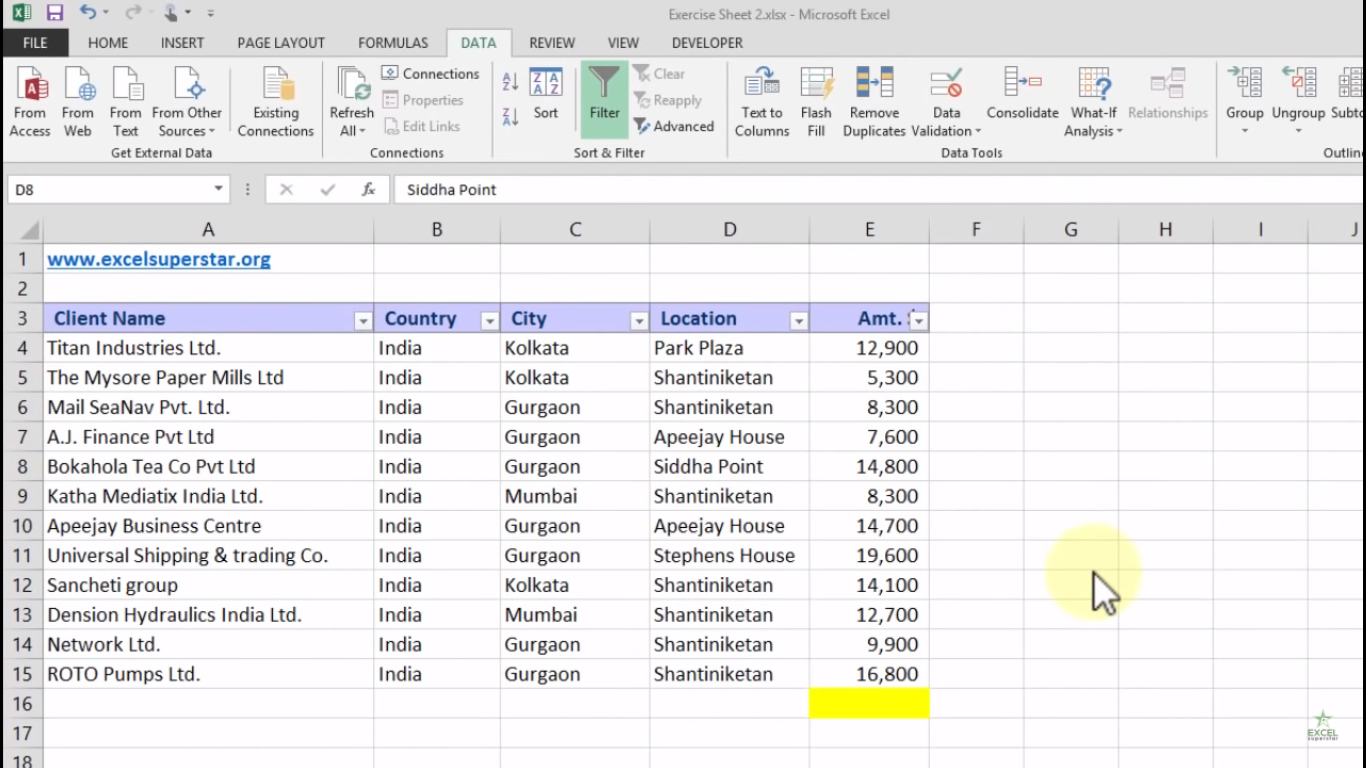2. Apply a Filter3. The SUM Function includes rows hidden by the filter

4. The SUBTOTAL function ignores rows hidden by a filter and calculates the correct result.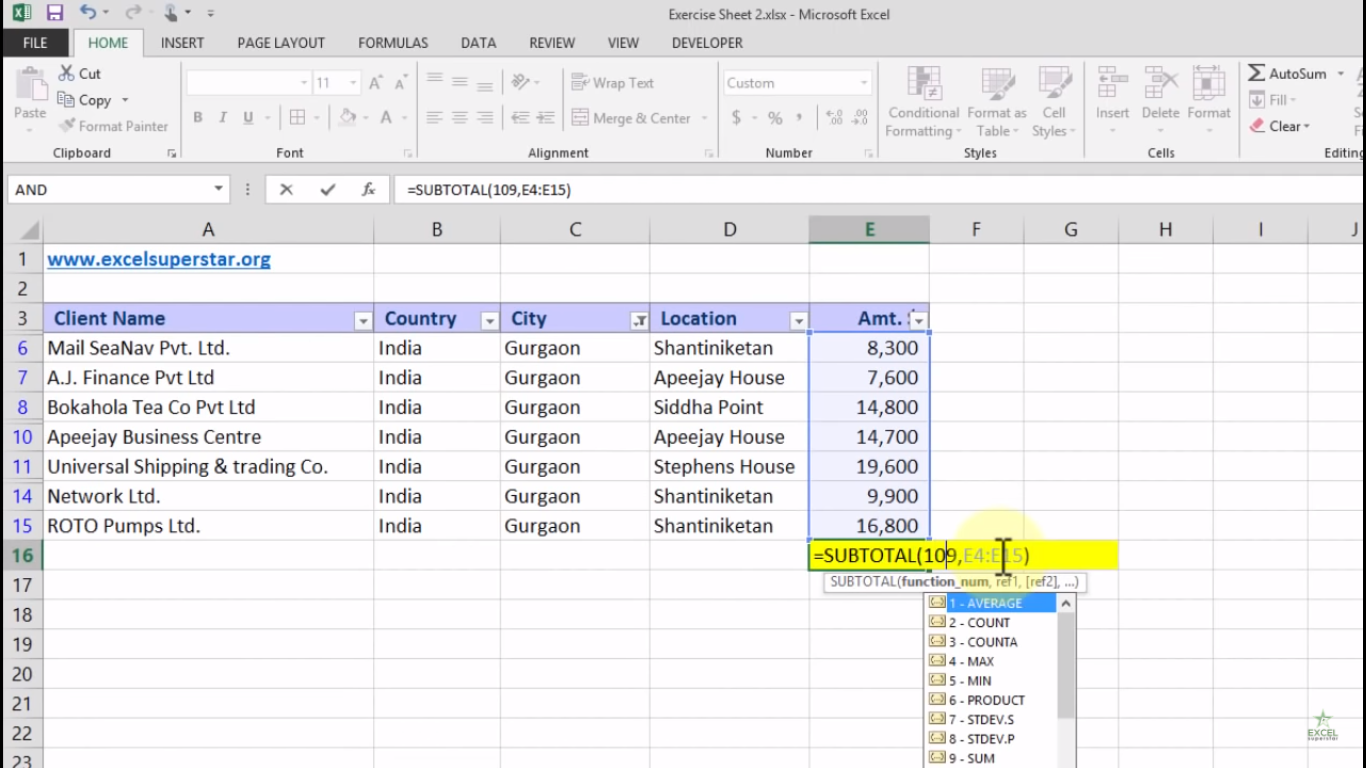Note: 109 is the argument for Sum if you use the SUBTOTAL function.

5. It is very confusing to remember which argument belongs to which function but Auto Complete feature helps you with this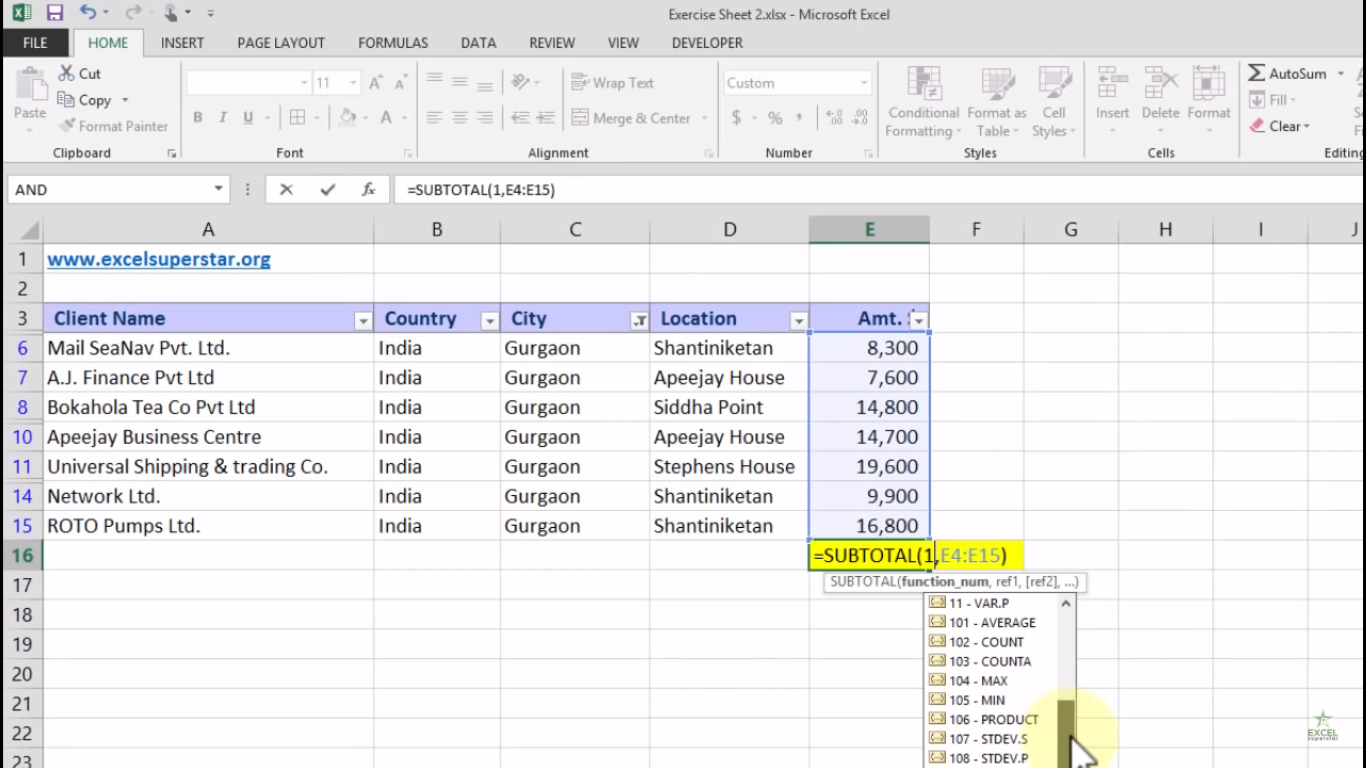Note – When filtering data, there’s no difference between the numbers 101-111 and the numbers 1-11. Using the numbers 101-111 is good because Excel uses these numbers in total rows at the end of tables.

2. Manually Hidden Rows

1. The SUM Function has below sums a range of cells in two columns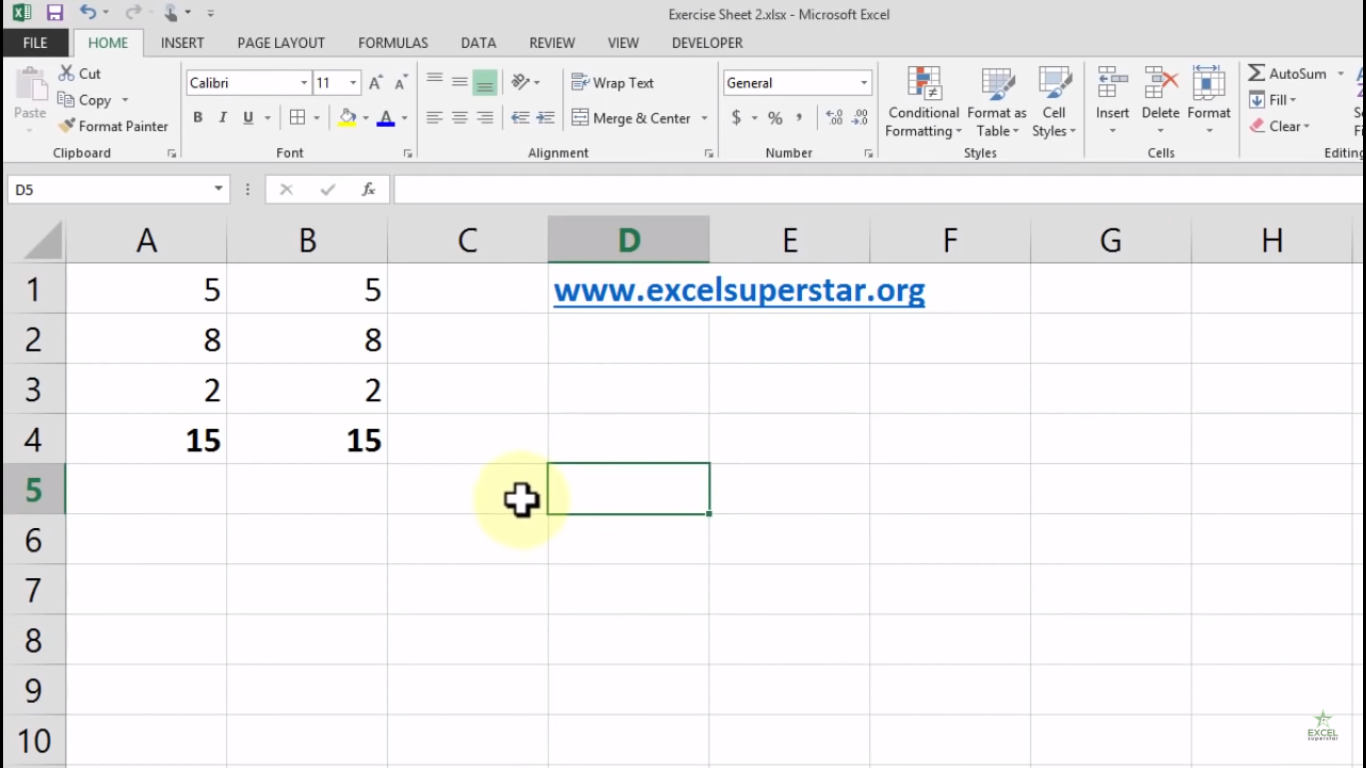2. Manually Hide Row 2 in both the columns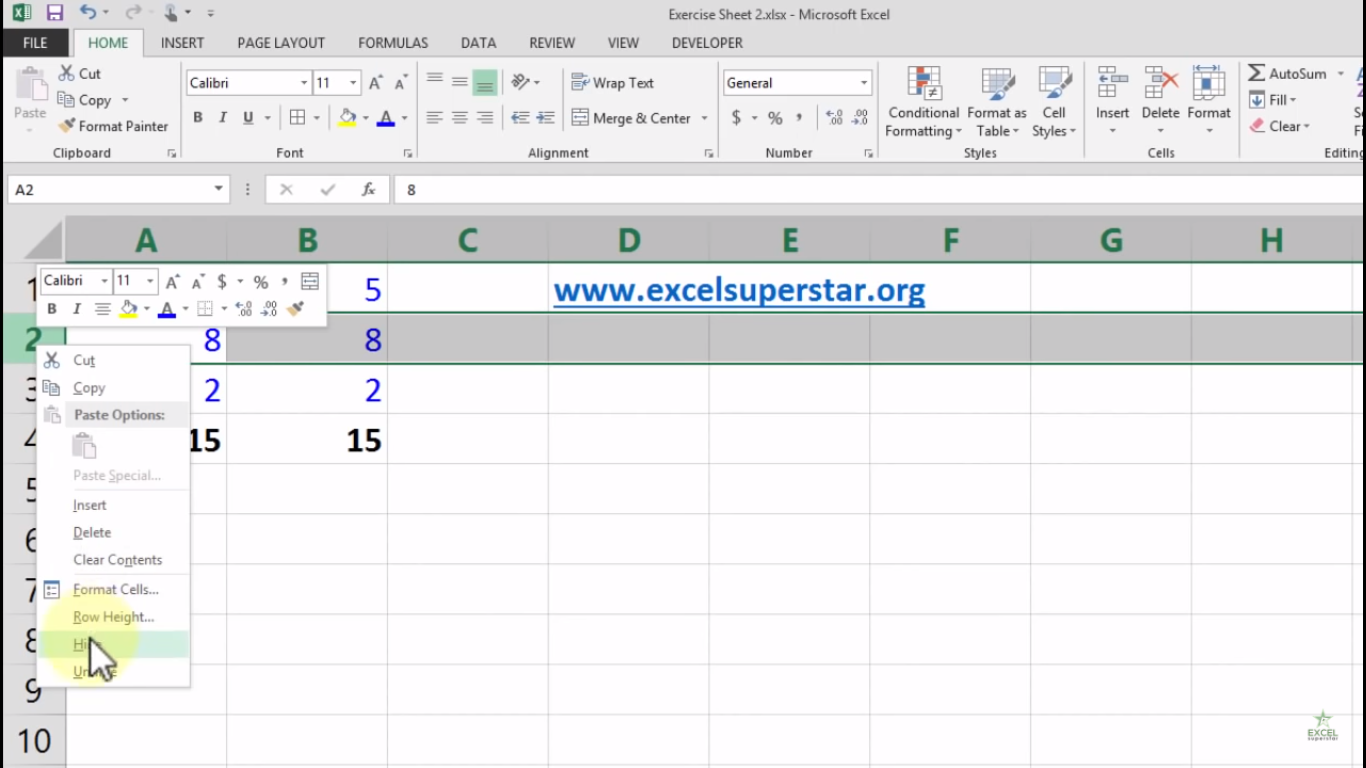3. The SUM Function includes manually hidden row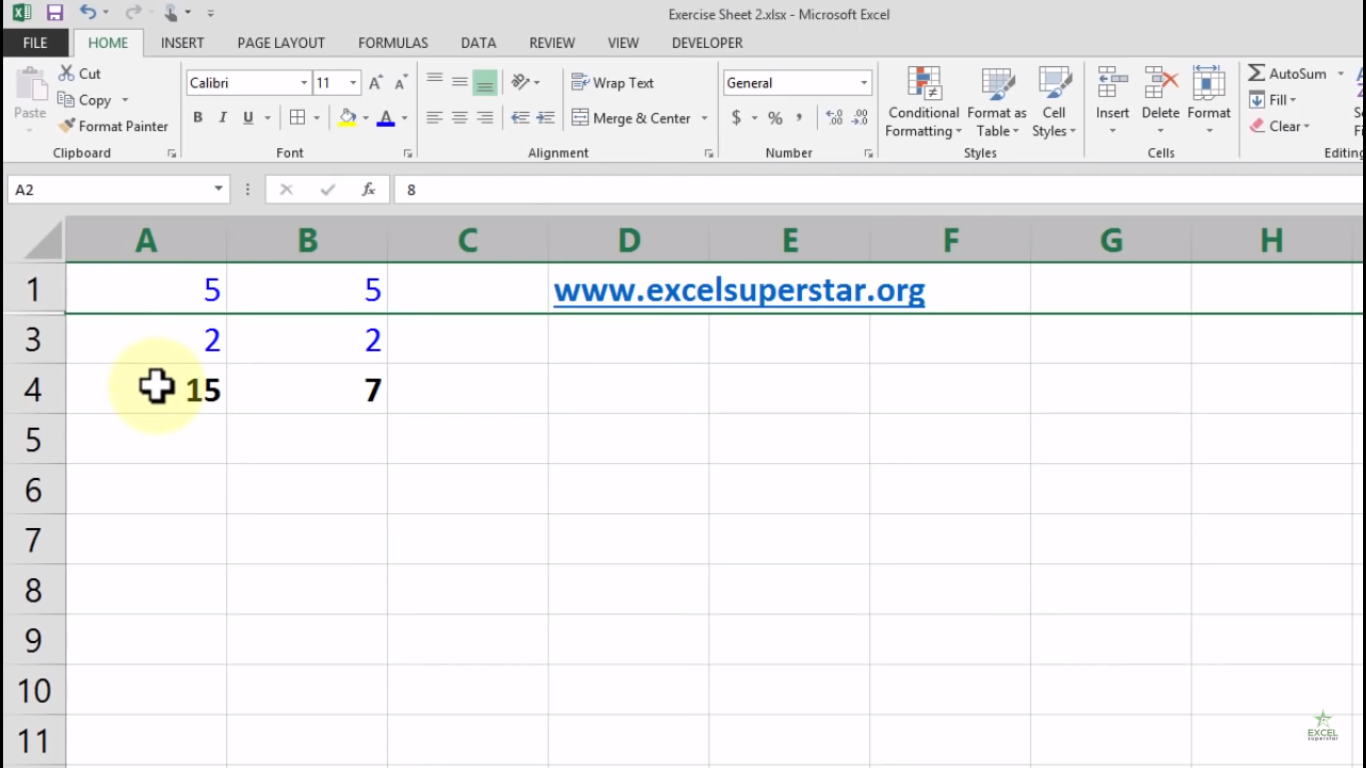4. The SUBTOTAL Function ignore manually hidden row and calculates the correct result